www.batmath.it

### Informal definition

The limit is the behaviour of a function f as x gets closer and closer to a given number c (or as x grows without bounds).

Despite this simple intuitive definition of a limit, the correct definition requires a lot of mathematics and is not simple at all to fully understand. We will not be interested in this: we'll only point out, from time to time, the problems that arise when one tries to formalize this concept. In this site you may find a more traditional treatment of this concept, in Italian.

Consider the following example.

Let D = {xR | x ≠ 0}, and consider the function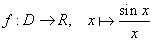(x is in radian, as usual when dealing with limits). We want to describe its behaviour as x approaches zero. Note that x=0 is not allowed for the function (sin0/0 does not make sense, and for this reason 0 is not in the domain). However it makes sense to ask: how does the function behave as x gets closer and closer to zero?.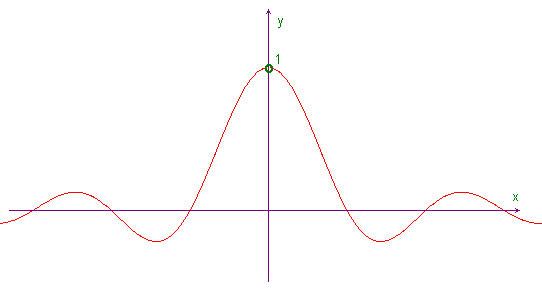The graph suggests that as x gets closer and closer to zero from either sides (and not reaching zero itself), f(x) approaches 1. This idea can be strengthened if we zoom in on the graph near the point (0,1). We may also use a spreadsheet to make numerical calculations and we'll always obtain the same result: as x gets closer and closer to zero from either sides the values of f approach 1. You can see a list of values obtained with Microsoft Excel.

The standard notation for this fact is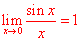. Sometimes we also write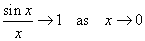.

Other examples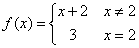As the following graph suggests we have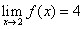, while f(2)=3.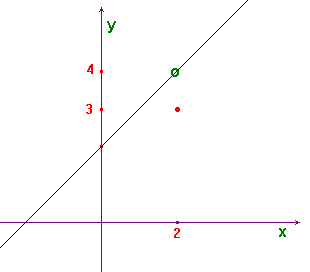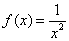From the graph we can deduce that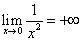.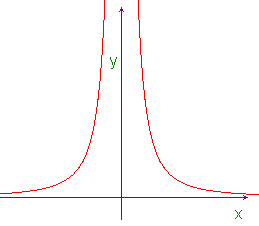From the same graph we can deduce that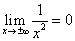.

Unfortunately the graph is not always so simple to interpret, due to obvious limitations in computer graphics. We consider now a most complicated function: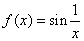. If we try to graph it we obtain something like the following picture, where we have drawn three different enlargements near the origin: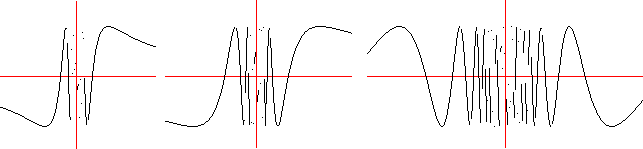As you can easily see the picture does not help us to understand what is the behaviour of the function when x approaches zero. In this case we must use other techniques to investigate the function's behaviour. For instance we can observe that, if we change the variable form x to 1/x, all points between 0 and 1 will have a corresponding point between 1 and +∞: we can interpret the graph of sin(1/x), between zero and one, as the compression of the graph of sinx between 1 and +∞. So to find the behaviour of sin(1/x) as x approaches zero it is sufficient to investigate the behaviour of sinx as x approaches +∞; this last behaviour is easy to figure: sinx fluctuates between -1 and 1 without approaching any definite value. We write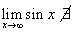or, in an equivalent manner,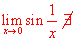.

It is important to observe that finding the behaviour of a function f as x gets closer and closer to a given number c makes sense only if in the neighbourhood of the point c, possibly not belonging to the domain of f, there are points of the domain of f, otherwise the values of the function cannot be calculated. We shall say that the point c must be an accumulation point for the domain of f: an accumulation point for a set is a point (possibly not belonging to the set) in whose neighbourhoods we can always find points of the set, no matter how the neighbourhood is small.

We can summarize all this in the following:

Informal definition

Suppose we are given a function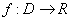, and an accumulation point c for D, then we say that the limit of f(x), as x approaches c, is l if f(x) gets arbitrary close to l, when x is sufficiently close to c

We write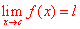or, equivalently,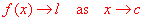.

first published on march 26 2002 - last updated on september 01 2003